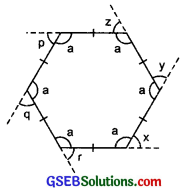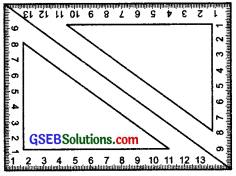# GSEB Solutions Class 8 Maths Chapter 3 Understanding Quadrilaterals InText Questions

Gujarat Board GSEB Textbook Solutions Class 8 Maths Chapter 3 Understanding Quadrilaterals InText Questions and Answers.

## Gujarat Board Textbook Solutions Class 8 Maths 3 Understanding Quadrilaterals InText Questions

Try These (Page 43)

Question 1 .
Take a regular hexagon as shown in the figure:

1. What is the sum of the measures of its exterior angles x, y, z, p, q, r?

2. Is x = y = z = p = q = r? Why?

3. What is the measure of each?(i) exterior angle
(ii) interior angle

4. Repeat this activity for the cases of
(i) A regular octagon
(ii) a regular 20-gon
Solution:
1. ∠x + ∠y + ∠z + ∠p + ∠q + ∠r = 360°
[∵ Sum of exterior angles of a polygon = 360°]

2. Since, all the sides of the polygon are equal.
∴ It is a regular hexagon.
So, its interior angles are equal.
x = (180° – a) y = (180° – a)
z = (180° – a) p = (180° – a)
q = (180° – a) r = (180° – a)
∴ x – y = z = p = q = r

3.
(i) ∵ x + y + z + p + q + r = 360°
[∵ sum of exterior angles = 360°] and all these angles are equal.
∴ Measure of each exterior angle
= $$\frac{360^{\circ}}{6}$$ = 60°

(ii) ∵ Exterior angle = 60°
∴ 180° – 60° = Interior angle
or 120°= Interior angle
or Measure of interior angle = 120°

4.
(i) In a regular octagon, number of sides (n) = 8
∴Each exterior angle = $$\frac{360^{\circ}}{8}$$ = 45°
∴Each interior angle = 180° – 45° = 135°

(ii) For a regular 20-gon, the number of sides (n) = 20
∴Each exterior angle = $$\frac{360^{\circ}}{20}$$ = 18°
Thus, each interior angle = 180° – 18° = 162°Question 2.
Find the number of sides of a regular polygon whose each exterior angle has a measure of 40°?
Solution:
Since, the given polygon is a regular polygon.
∴ Its each exterior angle is equal.
∵ Sum of all the exterior angles = 360°
∴ Number of exterior angles = $$\frac{360^{\circ}}{40^{\circ}}$$ = 9
⇒ Number of sides = 9
Thus, it is a nonagon.

Try These (Page 47)

Question 1.
Take two identical set squares with angles 30° – 60° – 90° and place them adjacently to form a parallelogram as shown in Figure? Does this help to you to verify the above property?Solution:
The given figure helps us to verify that Opposite sides of a parallelogram are of equal length.

Try These (Page 48)

Question 1.
Take two identical 30° – 60° – 90° set – squares and form a parallelogram as before. Does the figure obtained help you to confirm the above property?
Solution:
Above figure also help us to confirm that: opposite angles of a parallelogram are equal.

Try These (Page 50)

Question 1.
After showing m∠R = m∠N = 70°, can you find m∠I and m∠G by any other method?Solution:
Yes, without using the property of a parallelogram, we can also find m∠I and m∠G as given below:
∵ m∠R = m∠N = 70° and RG || IN. the transversal RI intersecting them.
∴ m∠R + m∠I = 180°
[Sum of interior opposite angles is 180°]
or 70° + m∠I = 180°
m∠I = 180° – 70° = 110°
Similarly, m∠G = 110°Question 2.
In the figure. ABCD is a parallelogram. Given that OD = 5 cm and AC is 2 cm less than BD. Find OA?Solution:
∵ Diagonals of a parallelogram bisect each other.
∴ OD = OB =5 cm
or OB = 5cm
or BD = 5 cm × 2 = 10 cm
∵ AC = BD – 2cm
∴ AC = (10 – 2) cm = 8 cm
0r $$\frac{1}{2}$$ AC = $$\frac{1}{2}$$ × 8 cm = 4cm
or OA = 4 cm.

Try These (Page 56)

Question 1.
A mason has made a concrete slab. He needs it to be rectangular In what different ways can he make sure thai it is rectangular?
Solution:
He can make sure thai it is rectangular using the following different ways:

1. By making opposite sides of equal length.
2. By keeping each angle at the corners as 900.
3. By keeping the diagonals of equal length.
4. By making opposite sides parallel and ensuring one angle as 900 in measure.
5. By making all angles equal and ensuring the measure of one angle as 900.Question 2.
A square was defined as a rectangle with all sides equal. Can we dfine it as rhombus with equal angles? Explore this idea?
Solution:
Yes, because a rhombus becomes a square if its all angles are equal.

Question 3.
Can a trapezium have all angles equal? Can it have all sides equal? Explain?
Solution:
Yes, if it is a rectangle. It can have all sides equal, when it becomes a square.